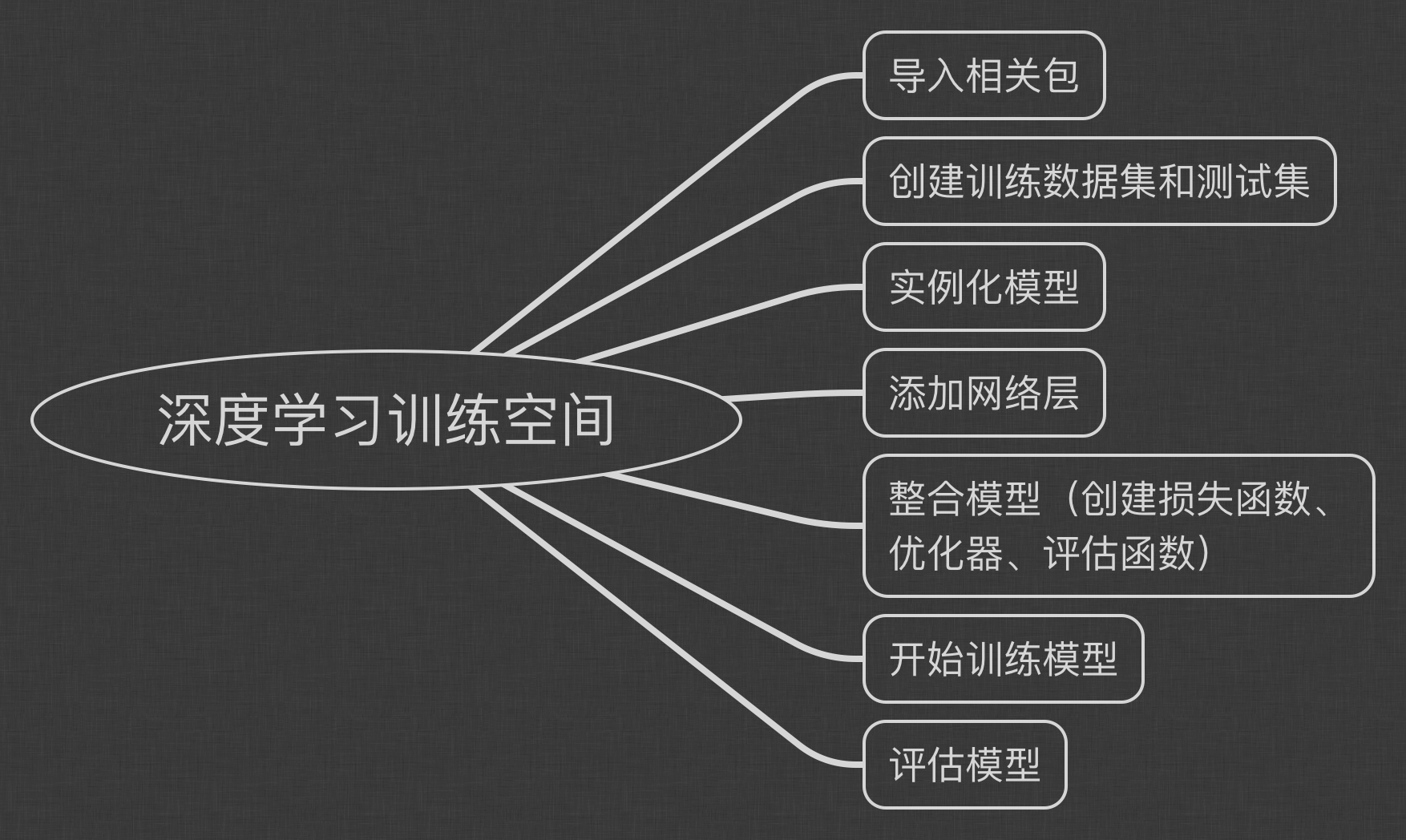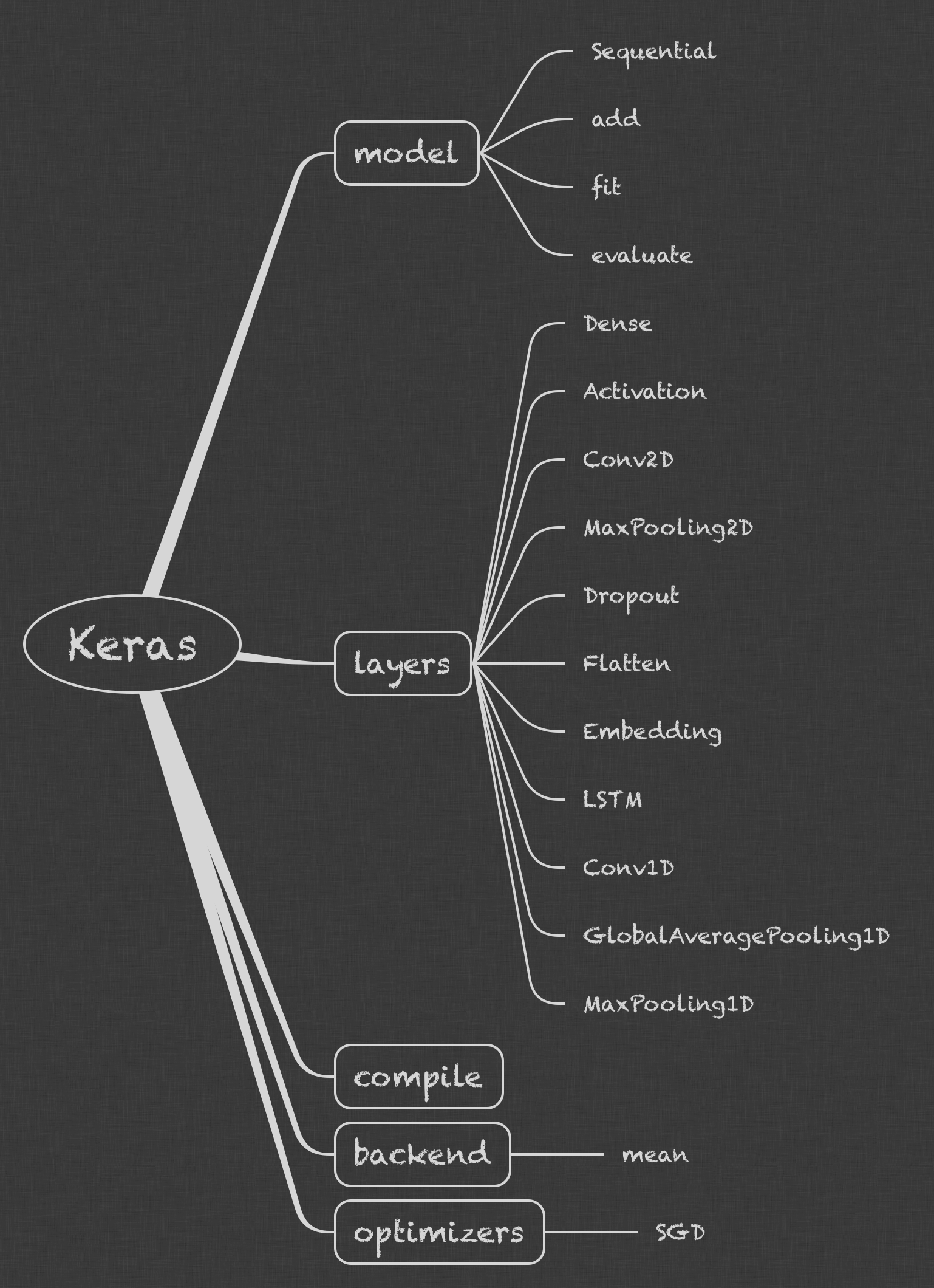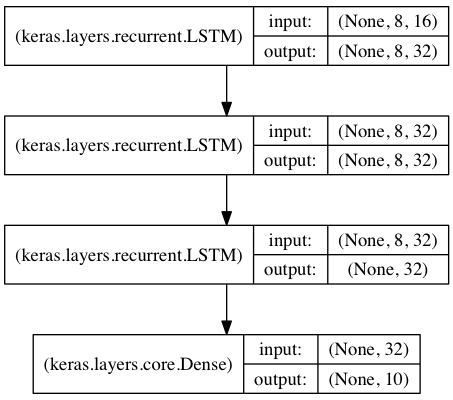2020-02-23 12:59:07 qq_43667130 阅读数 51
• ###### 三个项目玩转深度学习（附1G源码）

从事大数据与人工智能开发与实践约十年，钱老师亲自见证了大数据行业的发展与人工智能的从冷到热。事实证明，计算机技术的发展，算力突破，海量数据，机器人技术等，开启了第四次工业革命的序章。深度学习图像分类一直是人工智能的经典任务，是智慧零售、安防、无人驾驶等机器视觉应用领域的核心技术之一，掌握图像分类技术是机器视觉学习的重中之重。针对现有线上学习的特点与实际需求，我们开发了人工智能案例实战系列课程。打造：以项目案例实践为驱动的课程学习方式，覆盖了智能零售，智慧交通等常见领域，通过基础学习、项目案例实践、社群答疑，三维立体的方式，打造最好的学习效果。

609 人正在学习 去看看 钱兴会

## 第一次开始学习

https://www.bilibili.com/video/av36993857?from=search&seid=8894021963757644142

https://www.bilibili.com/video/av81430943from=search&seid=8894021963757644142

https://www.bilibili.com/video/av77638697?p=61

## 我认为应该有怎样的基础

1：首先编程基础要有吧，我大一玩单片机时学的C语言，之后学opencv计算机视觉学的C++，而在学《机器视觉实战》时巩固了一下python，虽然我编程不咋样，但一些基本功还是有的。

2：其次明确个方向，机器学习，深度学习可以应用的方面很多，而你如果单学不用，是不会有什么收获的，所以你需要知道你学了是想干什么，就像我是学计算机视觉的，而它本身就是深度学习一大应用领域。

## 我的深度学习环境的搭建

• caffe
• tensorflow
• pytorch

https://cuijiahua.com/

https://cuijiahua.com/blog/2019/11/dl-14.html

《动手学深度学习》

## 百度网盘

2017-10-16 15:15:28 Scythe666 阅读数 431
• ###### 三个项目玩转深度学习（附1G源码）

从事大数据与人工智能开发与实践约十年，钱老师亲自见证了大数据行业的发展与人工智能的从冷到热。事实证明，计算机技术的发展，算力突破，海量数据，机器人技术等，开启了第四次工业革命的序章。深度学习图像分类一直是人工智能的经典任务，是智慧零售、安防、无人驾驶等机器视觉应用领域的核心技术之一，掌握图像分类技术是机器视觉学习的重中之重。针对现有线上学习的特点与实际需求，我们开发了人工智能案例实战系列课程。打造：以项目案例实践为驱动的课程学习方式，覆盖了智能零售，智慧交通等常见领域，通过基础学习、项目案例实践、社群答疑，三维立体的方式，打造最好的学习效果。

609 人正在学习 去看看 钱兴会

1、深度学习的历史与基础知识

1.0 书籍

 Bengio, Yoshua, Ian J. Goodfellow, and Aaron Courville. 深度学习（Deep learning）, An MIT Press book. (2015). （这是深度学习领域的圣经，你可以在读此书的同时阅读下面的论文）。

1.1 调查类：

 LeCun, Yann, Yoshua Bengio, and Geoffrey Hinton. 深度学习 (Deep learning), Nature 521.7553 (2015): 436-444. (深度学习三位大牛对各种学习模型的评价)

1.2 深度信念网络（DBN）（深度学习前夜的里程碑）

 Hinton, Geoffrey E., Simon Osindero, and Yee-Whye Teh. 一个关于深度信念网络的快速学习算法（A fast learning algorithm for deep belief nets）, (深度学习的前夜)

 Hinton, Geoffrey E., and Ruslan R. Salakhutdinov. 使用神经网络降低数据的维度（Reducing the dimensionality of data with neural networks）, (里程碑式的论文，展示了深度学习的可靠性)

1.3 ImageNet 的演化（深度学习从这里开始）

 Krizhevsky, Alex, Ilya Sutskever, and Geoffrey E. Hinton. 使用深度卷积神经网络进行 ImageNet 分类任务（Imagenet classification with deep convolutional neural networks）(AlexNet, 深度学习的突破)

 Simonyan, Karen, and Andrew Zisserman. 针对大尺度图像识别工作的的超深卷积网络（Very deep convolutional networks for large-scale image recognition） (VGGNet, 神经网络开始变得非常深！)

 Szegedy, Christian, et al. 更深的卷积（Going deeper with convolutions）(GoogLeNet)

 He, Kaiming, et al. 图像识别的深度残差学习（Deep residual learning for image recognition）(ResNet，超级超级深的深度网络！CVPR--IEEE 国际计算机视觉与模式识别会议-- 最佳论文)

1.4 语音识别的演化

 Hinton, Geoffrey, et al. 语音识别中深度神经网络的声学建模（Deep neural networks for acoustic modeling in speech recognition: The shared views of four research groups）（语音识别中的突破)

 Graves, Alex, Abdel-rahman Mohamed, and Geoffrey Hinton. 用深度循环神经网络进行语音识别（Speech recognition with deep recurrent neural networks）(RNN)

 Graves, Alex, and Navdeep Jaitly. 面向端到端语音识别的循环神经网络（Towards End-To-End Speech Recognition with Recurrent Neural Networks）

 Sak, Ha?im, et al. 语音识别中快且精准的循环神经网络声学模型（Fast and accurate recurrent neural network acoustic models for speech recognition）(语音识别系统)

 Amodei, Dario, et al. Deep speech 2:英语和汉语的端到端语音识别（Deep speech 2: End-to-end speech recognition in english and mandarin）(百度语音识别系统)

 W. Xiong, J. Droppo, X. Huang, F. Seide, M. Seltzer, A. Stolcke, D. Yu, G. Zweig，在对话语音识别中实现人类平等（Achieving Human Parity in Conversational Speech Recognition）

2 深度学习方法

2.1 模型

 Hinton, Geoffrey E., et al. 通过避免特征检测器的共适应来改善神经网络（Improving neural networks by preventing co-adaptation of feature detectors）(Dropout)

 Srivastava, Nitish, et al. Dropout：一种避免神经网络过度拟合的简单方法（Dropout: a simple way to prevent neural networks from overfitting）

 Ioffe, Sergey, and Christian Szegedy. Batch normalization:通过减少内部协变量加速深度网络训练（Batch normalization: Accelerating deep network training by reducing internal covariate shift）(2015 年一篇杰出论文)

 Ba, Jimmy Lei, Jamie Ryan Kiros, and Geoffrey E. Hinton.层归一化（Layer normalization）(批归一化的升级版)

 Courbariaux, Matthieu, et al. 二值神经网络：训练神经网络的权重和激活约束到正 1 或者负 1（Binarized Neural Networks: Training Neural Networks with Weights and Activations Constrained to+ 1 or?1）(新模型，快)

 Chen, Tianqi, Ian Goodfellow, and Jonathon Shlens. Net2net：通过知识迁移加速学习（Net2net: Accelerating learning via knowledge transfer） (修改之前的训练网络以减少训练)

 Wei, Tao, et al. 网络形态（Network Morphism）(修改之前的训练网络以减少训练 epoch)

2.2 优化

 Sutskever, Ilya, et al. 有关深度学习中初始化与动量因子的研究（On the importance of initialization and momentum in deep learning） (动量因子优化器)

 Kingma, Diederik, and Jimmy Ba. Adam：随机优化的一种方法（Adam: A method for stochastic optimization）(可能是现在用的最多的一种方法)

 Andrychowicz, Marcin, et al. 通过梯度下降学习梯度下降（Learning to learn by gradient descent by gradient descent） (神经优化器，令人称奇的工作)

 Han, Song, Huizi Mao, and William J. Dally. 深度压缩：通过剪枝、量子化训练和霍夫曼代码压缩深度神经网络（Deep compression: Compressing deep neural network with pruning, trained quantization and huffman coding） (ICLR 最佳论文，来自 DeePhi 科技初创公司，加速 NN 运行的新方向)

 Iandola, Forrest N., et al. SqueezeNet：带有 50x 更少参数和小于 1MB 模型大小的 AlexNet-层级精确度（SqueezeNet: AlexNet-level accuracy with 50x fewer parameters and< 1MB model size.） (优化 NN 的另一个新方向，来自 DeePhi 科技初创公司)

2.3 无监督学习／深度生成模型

 Le, Quoc V. 通过大规模无监督学习构建高级特征（Building high-level features using large scale unsupervised learning.） (里程碑，吴恩达，谷歌大脑，猫)

 Kingma, Diederik P., and Max Welling. 自动编码变异贝叶斯（Auto-encoding variational bayes.） (VAE)

 Goodfellow, Ian, et al. 生成对抗网络（Generative adversarial nets.）(GAN, 超酷的想法)

 Radford, Alec, Luke Metz, and Soumith Chintala. 带有深度卷曲生成对抗网络的无监督特征学习（Unsupervised representation learning with deep convolutional generative adversarial networks.）(DCGAN)

 Gregor, Karol, et al. DRAW：一个用于图像生成的循环神经网络（DRAW: A recurrent neural network for image generation.） (值得注意的 VAE，杰出的工作)

 Oord, Aaron van den, Nal Kalchbrenner, and Koray Kavukcuoglu. 像素循环神经网络（Pixel recurrent neural networks.）(像素 RNN)

 Oord, Aaron van den, et al. 使用像素 CNN 解码器有条件地生成图像（Conditional image generation with PixelCNN decoders.） (像素 CNN)

2.4 RNN／序列到序列模型

 Graves, Alex. 带有循环神经网络的生成序列（Generating sequences with recurrent neural networks.）(LSTM, 非常好的生成结果，展示了 RNN 的力量)

 Cho, Kyunghyun, et al. 使用 RNN 编码器-解码器学习词组表征用于统计机器翻译（Learning phrase representations using RNN encoder-decoder for statistical machine translation.） (第一个序列到序列论文)

 Sutskever, Ilya, Oriol Vinyals, and Quoc V. Le. 运用神经网路的序列到序列学习（Sequence to sequence learning with neural networks.」）(杰出的工作)

 Bahdanau, Dzmitry, KyungHyun Cho, and Yoshua Bengio. 通过共同学习来匹配和翻译神经机器翻译（Neural Machine Translation by Jointly Learning to Align and Translate.）

 Vinyals, Oriol, and Quoc Le. 一个神经对话模型（A neural conversational model.）(聊天机器人上的序列到序列)

2.5 神经图灵机

 Graves, Alex, Greg Wayne, and Ivo Danihelka. 神经图灵机器（Neural turing machines.）arXiv preprint arXiv:1410.5401 (2014). (未来计算机的基本原型）

 Zaremba, Wojciech, and Ilya Sutskever. 强化学习神经图灵机（Reinforcement learning neural Turing machines.）

 Weston, Jason, Sumit Chopra, and Antoine Bordes. 记忆网络（Memory networks.）

 Sukhbaatar, Sainbayar, Jason Weston, and Rob Fergus. 端到端记忆网络（End-to-end memory networks.）

 Vinyals, Oriol, Meire Fortunato, and Navdeep Jaitly. 指示器网络（Pointer networks.）

 Graves, Alex, et al. 使用带有动力外部内存的神经网络的混合计算（Hybrid computing using a neural network with dynamic external memory.）(里程碑，结合上述论文的思想)

2.6 深度强化学习

 Mnih, Volodymyr, et al. 使用深度强化学习玩 atari 游戏（Playing atari with deep reinforcement learning.） (第一篇以深度强化学习命名的论文)

 Mnih, Volodymyr, et al. 通过深度强化学习达到人类水准的控制（Human-level control through deep reinforcement learning.） (里程碑)

 Wang, Ziyu, Nando de Freitas, and Marc Lanctot. 用于深度强化学习的决斗网络架构（Dueling network architectures for deep reinforcement learning.） (ICLR 最佳论文，伟大的想法 )

 Mnih, Volodymyr, et al. 用于深度强化学习的异步方法（Asynchronous methods for deep reinforcement learning.） (当前最先进的方法)

 Lillicrap, Timothy P., et al. 运用深度强化学习进行持续控制（Continuous control with deep reinforcement learning.） (DDPG)

 Gu, Shixiang, et al. 带有模型加速的持续深层 Q-学习（Continuous Deep Q-Learning with Model-based Acceleration.）

 Schulman, John, et al. 信赖域策略优化（Trust region policy optimization.） (TRPO)

 Silver, David, et al. 使用深度神经网络和树搜索掌握围棋游戏（Mastering the game of Go with deep neural networks and tree search.） (阿尔法狗)

2.7 深度迁移学习／终身学习／尤其对于 RL

 Bengio, Yoshua. 表征无监督和迁移学习的深度学习（Deep Learning of Representations for Unsupervised and Transfer Learning.） (一个教程)

 Silver, Daniel L., Qiang Yang, and Lianghao Li. 终身机器学习系统：超越学习算法（Lifelong Machine Learning Systems: Beyond Learning Algorithms.） (一个关于终生学习的简要讨论)

 Hinton, Geoffrey, Oriol Vinyals, and Jeff Dean. 提取神经网络中的知识（Distilling the knowledge in a neural network.） (教父的工作)

 Rusu, Andrei A., et al. 策略提取（Policy distillation.） (RL 领域)

 Parisotto, Emilio, Jimmy Lei Ba, and Ruslan Salakhutdinov. 演员模仿：深度多任务和迁移强化学习（Actor-mimic: Deep multitask and transfer reinforcement learning.） (RL 领域)

 Rusu, Andrei A., et al. 渐进神经网络（Progressive neural networks.）(杰出的工作，一项全新的工作)

2.8 一次性深度学习

 Lake, Brenden M., Ruslan Salakhutdinov, and Joshua B. Tenenbaum. 通过概率程序归纳达到人类水准的概念学习（Human-level concept learning through probabilistic program induction.）(不是深度学习，但是值得阅读)

 Koch, Gregory, Richard Zemel, and Ruslan Salakhutdinov. 用于一次图像识别的孪生神经网络（Siamese Neural Networks for One-shot Image Recognition.）

 Santoro, Adam, et al. 用记忆增强神经网络进行一次性学习（One-shot Learning with Memory-Augmented Neural Networks ） (一个一次性学习的基本步骤)

 Vinyals, Oriol, et al. 用于一次性学习的匹配网络（Matching Networks for One Shot Learning.）

 Hariharan, Bharath, and Ross Girshick. 少量视觉物体识别（Low-shot visual object recognition.）(走向大数据的一步)

3 应用

3.1 NLP（自然语言处理）

 Antoine Bordes, et al. 开放文本语义分析的词和意义表征的联合学习（Joint Learning of Words and Meaning Representations for Open-Text Semantic Parsing.）

 Mikolov, et al. 词和短语及其组合性的分布式表征（Distributed representations of words and phrases and their compositionality.） (word2vec)

 Sutskever, et al. 运用神经网络的序列到序列学习（Sequence to sequence learning with neural networks.）

 Ankit Kumar, et al. 问我一切：动态记忆网络用于自然语言处理（Ask Me Anything: Dynamic Memory Networks for Natural Language Processing.）

 Yoon Kim, et al. 角色意识的神经语言模型（Character-Aware Neural Language Models.）

 Jason Weston, et al. 走向人工智能-完成问题回答：一组前提玩具任务（Towards AI-Complete Question Answering: A Set of Prerequisite Toy Tasks.） (bAbI 任务)

 Karl Moritz Hermann, et al. 教机器阅读和理解（Teaching Machines to Read and Comprehend.）(CNN/每日邮件完形风格问题)

 Alexis Conneau, et al. 非常深度卷曲网络用于自然语言处理（Very Deep Convolutional Networks for Natural Language Processing.） (在文本分类中当前最好的)

 Armand Joulin, et al. 诡计包用于有效文本分类（Bag of Tricks for Efficient Text Classification.）(比最好的差一点，但快很多)

2020-03-14 13:49:46 weixin_39025679 阅读数 44
• ###### 三个项目玩转深度学习（附1G源码）

从事大数据与人工智能开发与实践约十年，钱老师亲自见证了大数据行业的发展与人工智能的从冷到热。事实证明，计算机技术的发展，算力突破，海量数据，机器人技术等，开启了第四次工业革命的序章。深度学习图像分类一直是人工智能的经典任务，是智慧零售、安防、无人驾驶等机器视觉应用领域的核心技术之一，掌握图像分类技术是机器视觉学习的重中之重。针对现有线上学习的特点与实际需求，我们开发了人工智能案例实战系列课程。打造：以项目案例实践为驱动的课程学习方式，覆盖了智能零售，智慧交通等常见领域，通过基础学习、项目案例实践、社群答疑，三维立体的方式，打造最好的学习效果。

609 人正在学习 去看看 钱兴会# 1 Keras 序列模型

## 1.1 添加网络层方法

from keras.models import Sequential
from keras.layers import Dense, Activation

model = Sequential([
Dense(32, input_shape=(784,)),
Activation('relu'),
Dense(10),
Activation('softmax'),
])


from keras.models import Sequential
from keras.layers import Dense, Activation

model = Sequential()#实例化序列模型


## 1.2 输入shape

model = Sequential()

model = Sequential()


## 1.3 合并Compilation

• optimize 优化器名称
• loss 损失函数
• metrics 评估方法

### 1.3.1 例子

# For a multi-class classification problem
model.compile(optimizer='rmsprop',
loss='categorical_crossentropy',
metrics=['accuracy'])

# For a binary classification problem
model.compile(optimizer='rmsprop',
loss='binary_crossentropy',
metrics=['accuracy'])

# For a mean squared error regression problem
model.compile(optimizer='rmsprop',
loss='mse')

# For custom metrics
import keras.backend as K

def mean_pred(y_true, y_pred):
return K.mean(y_pred)

model.compile(optimizer='rmsprop',
loss='binary_crossentropy',
metrics=['accuracy', mean_pred])


## 1.4 训练过程Training

kesra是基于Numpy数组

### 1.4.1对单一输入二分类深度学习框架

#### 1.4.1.1 初始化训练模型参数

# For a single-input model with 2 classes (binary classification):

model = Sequential()
model.compile(optimizer='rmsprop',
loss='binary_crossentropy',
metrics=['accuracy'])


#### 1.4.1.2 创建(加载)数据

# Generate dummy data
import numpy as np
data = np.random.random((1000, 100))
labels = np.random.randint(2, size=(1000, 1))


#### 1.4.1.3开始训练

# Train the model, iterating on the data in batches of 32 samples
model.fit(data, labels, epochs=10, batch_size=32)


### 1.4.2 对单一输入多分类分类深度学习框架（步骤与上面一致）

# For a single-input model with 10 classes (categorical classification):

model = Sequential()
model.compile(optimizer='rmsprop',
loss='categorical_crossentropy',
metrics=['accuracy'])

# Generate dummy data
import numpy as np
data = np.random.random((1000, 100))
labels = np.random.randint(10, size=(1000, 1))

# Convert labels to categorical one-hot encoding
one_hot_labels = keras.utils.to_categorical(labels, num_classes=10)

# Train the model, iterating on the data in batches of 32 samples
model.fit(data, one_hot_labels, epochs=10, batch_size=32)


# 2 一般深度学习训练过程框架

## 2.1 多层感知机对多分类的softmax 分类函数

### 2.1.1 导入包

import keras
from keras.models import Sequential
from keras.layers import Dense, Dropout, Activation
from keras.optimizers import SGD


### 2.1.2 加载数据

# Generate dummy data
import numpy as np
x_train = np.random.random((1000, 20))
y_train = keras.utils.to_categorical(np.random.randint(10, size=(1000, 1)), num_classes=10)
x_test = np.random.random((100, 20))
y_test = keras.utils.to_categorical(np.random.randint(10, size=(100, 1)), num_classes=10)



### 2.1.3 实例化模型并添加网络层

model = Sequential()
# Dense(64) is a fully-connected layer with 64 hidden units.
# in the first layer, you must specify the expected input data shape:
# here, 20-dimensional vectors.


### 2.1.4 整合模型（创建损失函数、优化器、评估函数）

sgd = SGD(lr=0.01, decay=1e-6, momentum=0.9, nesterov=True)
model.compile(loss='categorical_crossentropy',
optimizer=sgd,
metrics=['accuracy'])


### 2.1.5 开始训练

model.fit(x_train, y_train,
epochs=20,
batch_size=128)


### 2.1.6 评估训练结果

score = model.evaluate(x_test, y_test, batch_size=128)


## 2.2 多层感知机对二分类

import numpy as np
from keras.models import Sequential
from keras.layers import Dense, Dropout

# Generate dummy data
x_train = np.random.random((1000, 20))
y_train = np.random.randint(2, size=(1000, 1))
x_test = np.random.random((100, 20))
y_test = np.random.randint(2, size=(100, 1))

model = Sequential()

model.compile(loss='binary_crossentropy',
optimizer='rmsprop',
metrics=['accuracy'])

model.fit(x_train, y_train,
epochs=20,
batch_size=128)
score = model.evaluate(x_test, y_test, batch_size=128)


## 2.3 VGG-like convnet:可视化几何群网络

### 2.3.1 导入包

import numpy as np
import keras
from keras.models import Sequential
from keras.layers import Dense, Dropout, Flatten
from keras.layers import Conv2D, MaxPooling2D
from keras.optimizers import SGD


### 2.3.2 创建数据

# Generate dummy data
x_train = np.random.random((100, 100, 100, 3))
y_train = keras.utils.to_categorical(np.random.randint(10, size=(100, 1)), num_classes=10)
x_test = np.random.random((20, 100, 100, 3))
y_test = keras.utils.to_categorical(np.random.randint(10, size=(20, 1)), num_classes=10)


### 2.3.3 实例化模型

model = Sequential()


### 2.3.4 添加网络层

# input: 100x100 images with 3 channels -> (100, 100, 3) tensors.
# this applies 32 convolution filters of size 3x3 each.
model.add(Conv2D(32, (3, 3), activation='relu', input_shape=(100, 100, 3)))



### 2.3.5 整合模型（创建损失函数、优化器、评估函数）

sgd = SGD(lr=0.01, decay=1e-6, momentum=0.9, nesterov=True)
model.compile(loss='categorical_crossentropy', optimizer=sgd)


### 2.3.6 训练模型

model.fit(x_train, y_train, batch_size=32, epochs=10)


### 2.3.7 评估模型

score = model.evaluate(x_test, y_test, batch_size=32)


## 2.4 使用LSTM（长短期记忆网络）进行序列分类

from keras.models import Sequential
from keras.layers import Dense, Dropout
from keras.layers import Embedding
from keras.layers import LSTM

max_features = 1024

model = Sequential()

model.compile(loss='binary_crossentropy',
optimizer='rmsprop',
metrics=['accuracy'])

model.fit(x_train, y_train, batch_size=16, epochs=10)
score = model.evaluate(x_test, y_test, batch_size=16)


## 2.5 使用1-D卷积进行序列分类

from keras.models import Sequential
from keras.layers import Dense, Dropout
from keras.layers import Embedding
from keras.layers import Conv1D, GlobalAveragePooling1D, MaxPooling1D

seq_length = 64

model = Sequential()

model.compile(loss='binary_crossentropy',
optimizer='rmsprop',
metrics=['accuracy'])

model.fit(x_train, y_train, batch_size=16, epochs=10)
score = model.evaluate(x_test, y_test, batch_size=16)


## 2.6 使用LSTM堆进行序列分类from keras.models import Sequential
from keras.layers import LSTM, Dense
import numpy as np

data_dim = 16
timesteps = 8
num_classes = 10

# expected input data shape: (batch_size, timesteps, data_dim)
model = Sequential()
input_shape=(timesteps, data_dim)))  # returns a sequence of vectors of dimension 32
model.add(LSTM(32, return_sequences=True))  # returns a sequence of vectors of dimension 32
model.add(LSTM(32))  # return a single vector of dimension 32

model.compile(loss='categorical_crossentropy',
optimizer='rmsprop',
metrics=['accuracy'])

# Generate dummy training data
x_train = np.random.random((1000, timesteps, data_dim))
y_train = np.random.random((1000, num_classes))

# Generate dummy validation data
x_val = np.random.random((100, timesteps, data_dim))
y_val = np.random.random((100, num_classes))

model.fit(x_train, y_train,
batch_size=64, epochs=5,
validation_data=(x_val, y_val))


## 2.7 Same stacked LSTM model, rendered “stateful”

from keras.models import Sequential
from keras.layers import LSTM, Dense
import numpy as np

data_dim = 16
timesteps = 8
num_classes = 10
batch_size = 32

# Expected input batch shape: (batch_size, timesteps, data_dim)
# Note that we have to provide the full batch_input_shape since the network is stateful.
# the sample of index i in batch k is the follow-up for the sample i in batch k-1.
model = Sequential()
batch_input_shape=(batch_size, timesteps, data_dim)))

model.compile(loss='categorical_crossentropy',
optimizer='rmsprop',
metrics=['accuracy'])

# Generate dummy training data
x_train = np.random.random((batch_size * 10, timesteps, data_dim))
y_train = np.random.random((batch_size * 10, num_classes))

# Generate dummy validation data
x_val = np.random.random((batch_size * 3, timesteps, data_dim))
y_val = np.random.random((batch_size * 3, num_classes))

model.fit(x_train, y_train,
batch_size=batch_size, epochs=5, shuffle=False,
validation_data=(x_val, y_val))


# 3 源网址

https://keras.io/getting-started/sequential-model-guide/2015-06-11 10:44:46 dcxhun3 阅读数 855
• ###### 三个项目玩转深度学习（附1G源码）

从事大数据与人工智能开发与实践约十年，钱老师亲自见证了大数据行业的发展与人工智能的从冷到热。事实证明，计算机技术的发展，算力突破，海量数据，机器人技术等，开启了第四次工业革命的序章。深度学习图像分类一直是人工智能的经典任务，是智慧零售、安防、无人驾驶等机器视觉应用领域的核心技术之一，掌握图像分类技术是机器视觉学习的重中之重。针对现有线上学习的特点与实际需求，我们开发了人工智能案例实战系列课程。打造：以项目案例实践为驱动的课程学习方式，覆盖了智能零售，智慧交通等常见领域，通过基础学习、项目案例实践、社群答疑，三维立体的方式，打造最好的学习效果。

609 人正在学习 去看看 钱兴会

0、Andrew Ng的机器学习前四章的内容(线性回归与逻辑回归)

(中文版对应网易公开课v.163.com/special/openc)

1、CMU Advanced Introduction to Machine Learning PPT: cs.cmu.edu/~epxing/Clas

CAFFE是一个常用的deep learning的软件工具，其页面上有deep learning的tutorial材料，同时想要深入了解具体实现的话可以直接阅读源代码

2017-07-05 19:49:07 jiaxiaoit 阅读数 448
• ###### 三个项目玩转深度学习（附1G源码）

从事大数据与人工智能开发与实践约十年，钱老师亲自见证了大数据行业的发展与人工智能的从冷到热。事实证明，计算机技术的发展，算力突破，海量数据，机器人技术等，开启了第四次工业革命的序章。深度学习图像分类一直是人工智能的经典任务，是智慧零售、安防、无人驾驶等机器视觉应用领域的核心技术之一，掌握图像分类技术是机器视觉学习的重中之重。针对现有线上学习的特点与实际需求，我们开发了人工智能案例实战系列课程。打造：以项目案例实践为驱动的课程学习方式，覆盖了智能零售，智慧交通等常见领域，通过基础学习、项目案例实践、社群答疑，三维立体的方式，打造最好的学习效果。

609 人正在学习 去看看 钱兴会

## 深度学习–caffe试水

### 人工神经网络

1.人工神经元模型

2.BP神经网络

zk=f1(ni=0vkixi)$z_k = f_1(\sum_{i=0}^nv_{ki}x_i)$

yj=f2(pk=0wjkzk)$y_j=f_2(\sum_{k=0}^pw_{jk}z_k)$
f1f2$f_1和f_2$是单调上升函数，而且必须是有界函数，因为细胞传递的信号不能是无线增加的，必须有最大值！！！

v$v$个样本的误差Ev$E_v$:
Ev=12mj=1(tvjyvj)2$E_v = \frac{1}{2}\sum_{j=1}^m(t_j^v-y_j^v)^2$

E=12Pv=1mj=1(tvjyvj)2=Pv=1Ev)$E = \frac{1}{2}\sum_{v=1}^{P}\sum_{j=1}^{m}(t_j^v-y_j^v)^2 =\sum_{v=1}^{P}Ev)$
3.卷积神经网络

#### <一>LeNet网络

input->convolutions->subsampling->convolutions->subsampling->full connection->full connection->gaussian connections

xi=f(ai)=Atanh(sai)$x_i=f(a_i)=A*tanh(s_{a_i})$

yi=j(xjwij)2$y_i=\sum_j(x_j-w_{ij})^2$

LeNet的网络定义在lenet_train_test.prototxt文件中

./build/tools/caffe train –solver=examples/mnist/lenet_solver.prototxt
solver.prototxt是参数配置文件./build/tools/caffe.bin test -model=examples/mnist/lenet_train_test.prototxt -weights=examples/mnist/lenet_iter_10000.caffemodelok!先到这里吧，后续再更新。。。